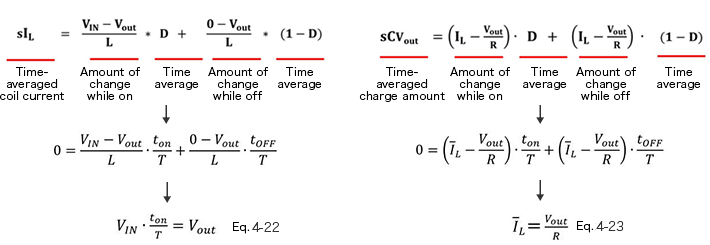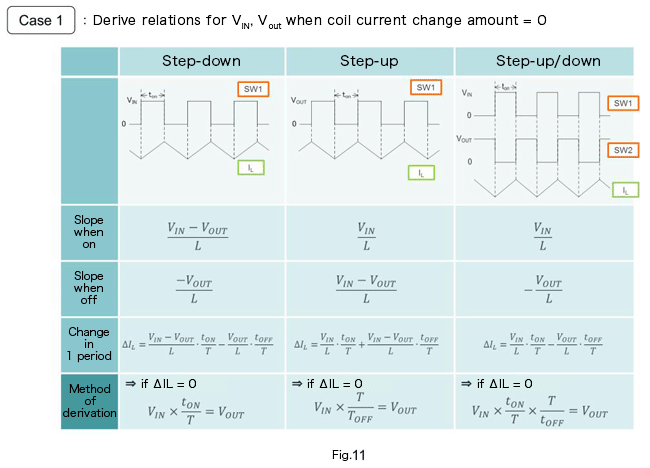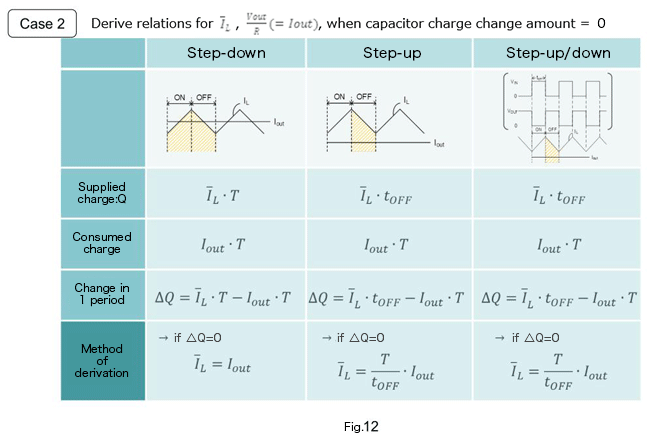# State-Space Averaging Method － Static Analysis

2017.08.24

・Static analysis is performed by substituting 0 for the static state into equations derived by state averaging.

・The results of static analysis can be applied to all modes, whether step-up, step-down, or step-up/down.

In the previous section, we explained “state-space averaging” in order to derive a transfer function that could be applied to step-down, step-up, and step-up/down modes. This time, we perform a static analysis based on the derived equations. The equations of the previous section are used, and so it may aid understanding to read the contents of the previous section as well.

## Static Analysis

The equations derived the previous time for coil current and capacitor charge amount are shown on the first line.

We perform a static analysis based on these equations. In a static analysis (steady state/stable state), the amount of change in the coil current and the amount of change in the charge on the output capacitor are both 0 (zero), and therefore the equations with 0 substituted are shown on the second line. D is rewritten as the time.

Rearranging these equations, we obtain equations 4-22 and 4-23 on the third line.Equation 4-22 is the well-known relation betweenVIN, Vout and D.

Equation 4-23 means that the average value of the coil current is supplied to the output load in one period.

This approach can be applied not only to step-down converters, but to step-up converters and step-up/down converters as well.

Below, static-state operation of the different converter types is summarized.